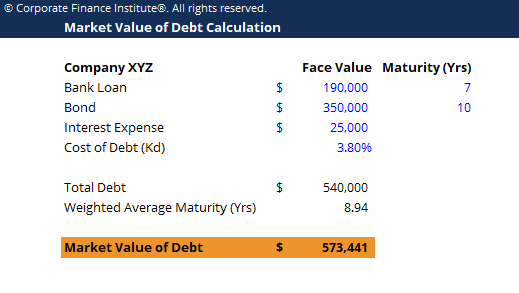# Market Value of Debt Template

## Market Value of Debt Template

This market value of debt template shows you how to calculate the market value of debt given the FV of total debt, interest expense, cost of debt and weighted average maturity.

Below is a preview of the market value of debt template:### Market Value of Debt Template

The market value of debt refers to the market price investors would be willing to buy a company’s debt at, which differs from the book value on the balance sheet. To estimate the market value of debt, an analyst can think of the total debt on the books as a single coupon bond, with the coupon being equal to the interest expenses on all debt and the maturity as the weighted average maturity of the debt.

The bond pricing formula to calculate market value of debt is:

C[(1 – (1/((1 + Kd)^t)))/Kd] + [FV/((1 + Kd)^t)]

Where C is the interest expense (in dollars)

Kd is the current cost of Debt (in percentages)

T is the weighted average maturity (in years)

FV represents the total debt

### More Free Templates

For more resources, check out our business templates library to download numerous free Excel modeling, PowerPoint presentation and Word document templates.

• Excel Modeling Templates
• PowerPoint Presentation Templates
• Transaction Document Templates

### Financial Analyst Certification

Become a certified Financial Modeling and Valuation Analyst (FMVA)® by completing CFI’s online financial modeling classes and training program!